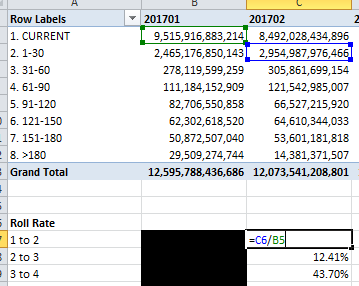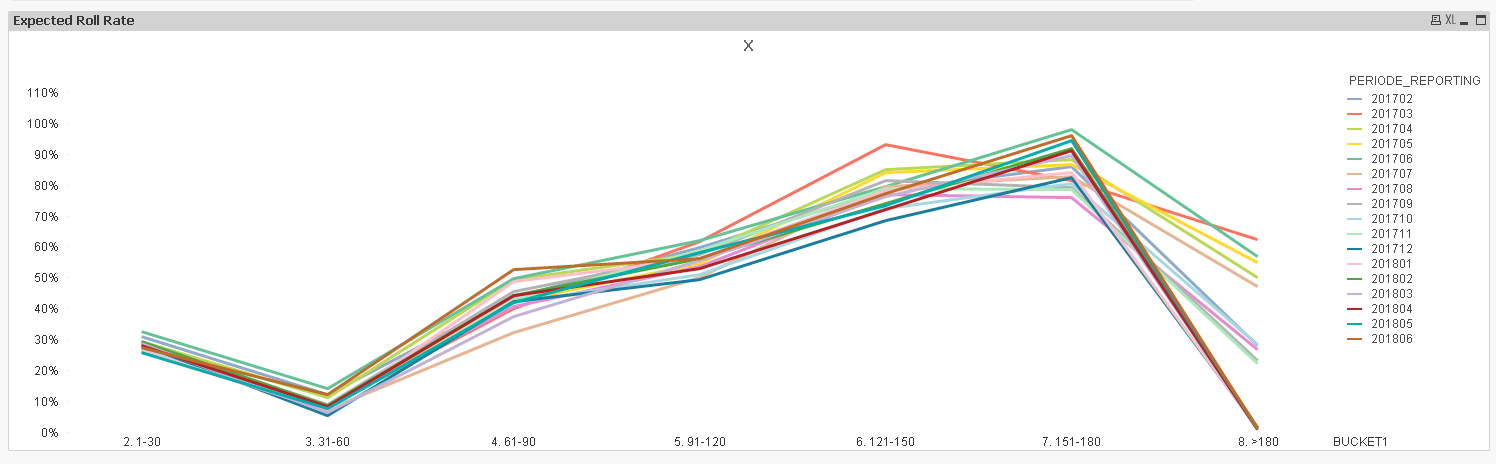Announcements
You can succeed best and quickest by helping others to succeed. Join the conversation.
cancel
Showing results for
Did you mean:Anonymous
Not applicable

## Need Help to create roll rate line chart from above and before function

Hi All,

Basically, I want to create Roll rate/Flow rate/Delinquency rate from my data.

It was very easy on Excel (as attached sample), but on qlikview I was only able to show the flow rate number correctly using pivot chart

with expressions:

=sum(TotalOSPrincipal)/above(before(SUM(TotalOSPrincipal)))

when I convert the pivot chart, it show "no data to display", I think above and before function is not suitable for this case, any idea or alternative to get the line chart?

FYI, to get the flow rate number I use formula as below :then easily create line chart based on roll rate number.

1 Solution

Accepted SolutionsMVP

Try this expression

=Sum(TotalOSPrincipal)/Aggr(Above(Aggr(Above(Sum(TotalOSPrincipal)), BUCKET1, PERIODE_REPORTING)), PERIODE_REPORTING, BUCKET1)5 RepliesMVP

Try this expression

=Sum(TotalOSPrincipal)/Aggr(Above(Aggr(Above(Sum(TotalOSPrincipal)), BUCKET1, PERIODE_REPORTING)), PERIODE_REPORTING, BUCKET1)MVP

Or this

=Sum(TotalOSPrincipal)/Only({<BUCKET1, PERIODE_REPORTING>}Aggr(Above(Only({<BUCKET1, PERIODE_REPORTING>}Aggr(Above(SUM({<BUCKET1, PERIODE_REPORTING>}TotalOSPrincipal)), BUCKET1, PERIODE_REPORTING))), PERIODE_REPORTING, BUCKET1))Anonymous
Not applicable
Author

Hi Sunny,

Thank You very much, it's work flawlessly!

I took so many time to resolve this using set analysis, but suddenly you solved this using Aggregation and above function.

I should learn again about those function, to know better when to use it.

Have a nice day...MVP

Just remember that set analysis is evaluated once per chart, so it can't be used for scenarios like these where you expecting a different value from set analysis every dimension. In these scenarios, Inter-Record Functions come in handyContributor II

Sunny.

I'm trying in qlik sense this same expression, because i'm with the same problem to calculate the roll rate.

But it's not working... I tryed this: